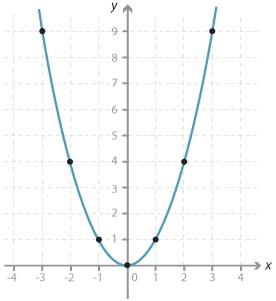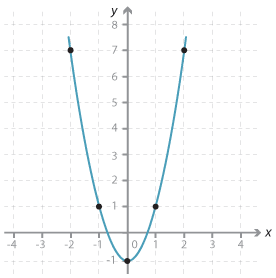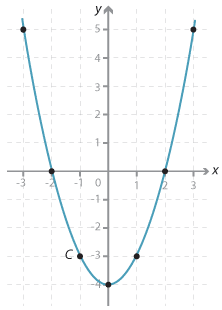## Content description

Graph simple non-linear relations with and without the use of digital technologies and solve simple related equations (ACMNA296)

Source: Australian Curriculum, Assessment and Reporting Authority (ACARA)

Consider the relationship given by y = x$$^2$$. This is not a linear relationship because x is raised to the power 2. First, we complete a table of values.

 $$x$$ $$y = x^2$$ −3 −2 −1 0 1 2 3 9 4 1 0 1 4 9

We then plot the corresponding points and join them with a smooth curve to obtain the graph below:Detailed description

This graph is called a parabola. The point (0, 0) is called the vertex or turning point of the parabola. The vertex corresponds to the minimum value of y, since x$$^2$$ is positive except when x = 0.

The graph is symmetrical about the y-axis. This line is called the axis of symmetry.

#### Example 1

Plot the graph of y = 2 x$$^2$$ − 1 for −2 ≤ x ≤ 2. Find the axis of symmetry and the coordinates of the vertex.

#### Solution

The table of values is shown:

 $$x$$ $$y = 2 x^2 - 1$$ –2 –1 0 1 2 7 1 –1 1 7Detailed description

Notice that the y-values are the same for x = −1 and x = 1. The equation of the axis of symmetry is x = 0. When x = 0, y = −1, so the vertex has coordinates (0, −1).

#### Example 2

Plot the graph of y = x$$^2$$ − 4 for −3 $$\le$$ x $$\le$$ 3. Give the coordinates of the vertex, the equation of the axis of symmetry and the coordinates of the x-intercepts and the y-intercept.

#### Solution

The table of values is shown:

 $$x$$ $$y = x^2 - 4$$ −3 −2 −1 0 1 2 3 5 0 −3 −4 −3 0 5Detailed description

The vertex has coordinates (0, −4).

The equation of axis of symmetry is x = 0.

The coordinates of the y-intercept are (0, −4).

The coordinates of the x-intercepts are (−2, 0) and (2, 0).